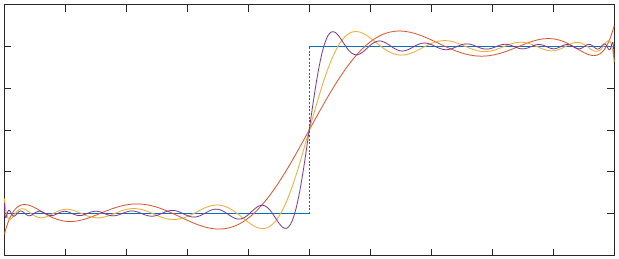Least squares approximation of sign(x) on [-1,1] with polynomials of degree 10,20,40

## Course information

• Since Fall 2015 the course AMSC 666 has a new syllabus, combining the most important topics of the former AMSC666, AMSC667. The goal was to make this course more attractive:
• students who only want to take one graduate numerical analysis course get to see more relevant topics
• students who are interested in topics like numerical PDE methods can more quickly take courses like AMSC714, AMSC715
• The AMSC qualifying exam rules were updated accordingly: The sequences
AMSC666, AMSC714 and AMSC666, AMSC715
were added to the list of permitted 2-course sequences
• AMSC/CMSC 466 (Introduction to Numerical Analysis) is a prerequisite for this course.
You should be familiar with the basic topics of numerical methods:
• The idea of AMSC/CMSC666 is to build on these results and cover more advanced techniques and theory. I will give a brief review of the AMSC466 results which we use in this class.
• I recommend the following book:
E. Süli: "An Introduction to Numerical Analysis", Cambridge University Press, 2003
Chapters 1,2,6,7 in this book contain the prerequisites for this course.
Chapters 8,9,10,12,13,14 are related to the material I want to cover in this course.

## Topics

• Approximation Theory (3 weeks, [1,2,3])
• Vector, Matrix and Functional Norms
• Least Squares, QR, SVD
• Orthogonal Polynomials
• Chebyshev Expansions
• Gaussian Quadrature
• Numerical Solution of Initial-Value Problems (3 weeks, [4,5,6])
• Consistency, Stability, and Convergence Analysis
• One-Step Methods, Runge-Kutta Methods
• Multistep Methods
• Methods for Stiff Problems
• Error Estimation and Adaptivity
• Iterative Methods for Linear Algebraic Systems (3 weeks, [7,8,9,10])
• Motivation: Boundary-Value Problems for Elliptic PDEs
• Classic Iterative Methods: Jacobi, Gauss-Seidel, SOR methods
• Conjugate Gradient Method and Preconditioning
• GMRES
• Optimization (3 weeks, [9,10,11])
• Steepest Descent, Newton and Quasi-Newton Methods
• Line Search Methods and Trust Region Methods
• Rates of Convergence
• Nonlinear Conjugate Gradient Method
• Nonlinear Least Squares Problem

## Literature

1. Lloyd N. Trefethen, Approximation Theory and Approximation Practice, SIAM 2012
first six chapters, Errata
2. James Demmel, Applied Numerical Linear Algebra, SIAM, 1997
freely available online via UMD Library
3. Rivlin, T. J., an Introduction to the Approximation of Functions, Dover, 1969
4. Gil, A., Segura, J., Temme, N., Numerical Methods for Special Functions, SIAM, 2007
chapter on Chebyshev Expansions is freely available online
5. Hairer, E., Norsett, S.P., Wanner, G., Solving Ordinary Differential Equations I. Nonstiff Problems, Second Revised Edition, Springer 1993
6. Hairer, E., Wanner, G., Solving Ordinary Differential Equations II. Stiff and Differential-Algebraic Problems, Second Revised Edition, Springer 1993
7. Deuflhard, P., Bornemann, F., Scientific Computing with Ordinary Differential Equations, Springer, 2002
8. Elman, H., Silvester, D., Wathen, A., Finite Elements and Fast Iterative Solvers, Second Edition, Oxford Science Publications, 2014
9. Morton, K. W., Mayers, D.F., Numerical Solutions of Partial Differential Equations, Second Edition, Cambridge, 2005
10. Nocedal, J., Wright, S., Numerical Optimization, Second Edition, Springer 2006
freely available online via UMD Library
11. Kelley, C. T., Iterative Methods for Linear and Nonlinear Equations, SIAM 1995
12. Kelley, C. T., Iterative Methods for Optimization, SIAM 1999
freely available online via UMD Library

## Matlab programming

We will use Matlab to see how various algorithms perform (or fail).
Matlab can be downloaded for free from TERPware.

How to hand in Matlab results for homeworks:

• You have to write an m-file for each problem. (Typing commands at the >> prompt and printing this out is not allowed)
• You have to hand in the m-file together with the output and graphics.
The easiest way is to use the publish command in Matlab (if you call additional m-files, you have to print them out separately).
• How to use the publish command in Matlab:
Example 1: Using publish with the m-file sample.m generates sample.html (which you can print out and hand in).
Example 2: this m-file shows how to format each problem. This is the generated output.
More information about publishing markup